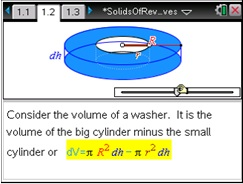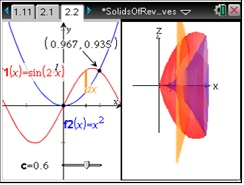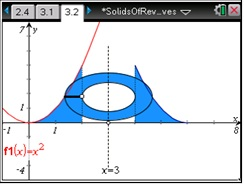# Activities

••• ##### Subject Area

• Math: Calculus: Definite Integrals and Applications

• ##### Author9-12

45 Minutes

• ##### Device
•TI-Nspire™ CX/CX II
•TI-Nspire™ CX CAS/CX II CAS
• TI-Nspire™
• TI-Nspire™ CAS
• ##### Software

TI-Nspire™
TI-Nspire™ CAS

3.2

## Solids Of Revolution Between Two Curves

#### Activity Overview

Students will investigate 3D visualizations of volumes created by rotating two functions about the x-or y-axis. They will understand the concept and reason for the volume formula in order to be prepared for generalizations. Students will solve the definite integral by hand using the fundamental theorem of calculus and using the definite integral capabilities of the TI-Nspire. Students will also explore find the volume of a shape between two lines rotated about a vertical line.

#### Key Steps

•First, students will explore the cross section of a region bounded by two curves rotated about a vertical line. They will then look at the difference between rotating functions about a vertical line versus as horizontal line.

•In the second problem, students will calculate the volume of a solid in which the function sin(2x) and another function have been rotated about the x-axis. They can use the slider at the bottom of the page to change the value of c, the location of the slice.

•Finally in the last problem, students will explore two functions rotated about a vertical line that is not the y-axis. An interactive page on page 3.2 allows students to change the line of rotation and the inner radius.